#### Quadratic denoising - House with hole (inpainting)

```Image size: 256 x 256, labels: 256
Data term: squared differences, except in hole where data costs = 0 for all labels
Smoothness term: squared differences (L2 norm), lambda=5
```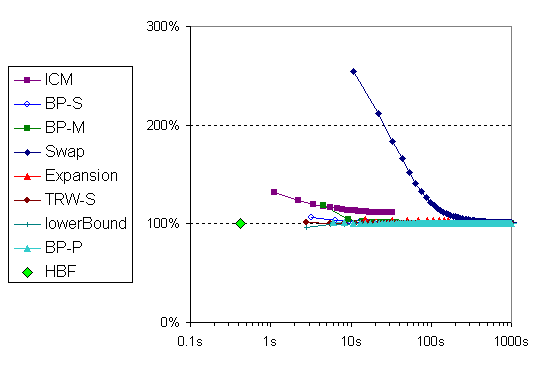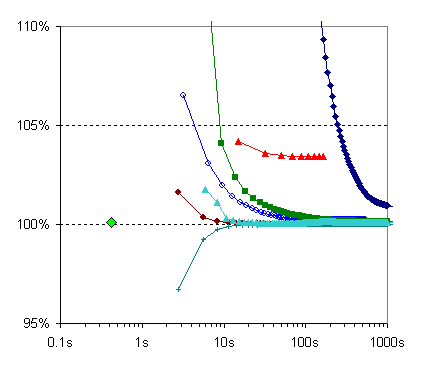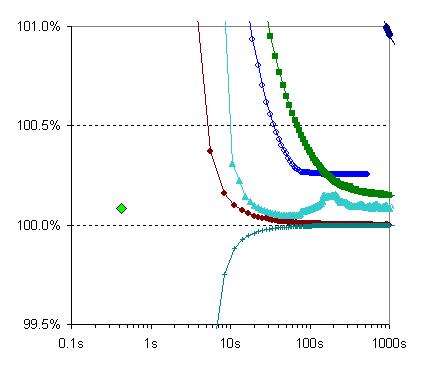BP-P: Pedro Felzenszwalb's BP implementation [P. Felzenszwalb and D. Huttenlocher, Efficient belief propagation for early vision, IJCV 70(1), 2006].

HBF: Rick Szeliski's fast quadratic solver using hierarchical basis functions [R. Szeliski, Locally adapted hierarchical basis preconditioning, SIGGRPAH 2006].

Since this is a quadratic problem, there is a single (real-valued) global minimum, which can be found with a quadratic solver. If ICM was run with real-valued variables, it would converge to this solution. For comparison, the plots contain the solution obtained after 5 iterations of the (HBF) solver, which takes less than 0.5 seconds. The solution, when rounded to the nearest integers, has an energy about 0.1% higher than the best integer solution found by TRW-S.

Input image (noise contaminated), and the original image ("ground truth"):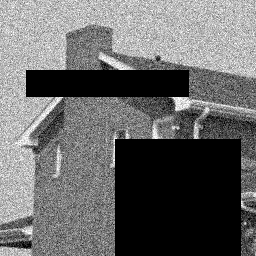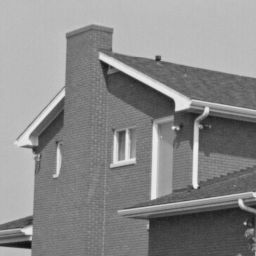(The black areas have data costs = 0 for all labels.)

All algorithms use the input intensities as start values.

Result images and their energies: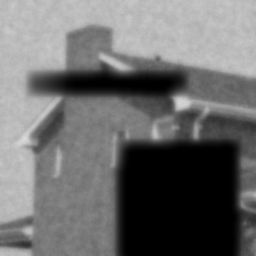max lower bound 37,580,519.6 ICM 41,813,618 (Ed= 31205618, Es= 10608000) BP-S 37,676,160 (Ed= 30679350, Es= 6996810) BP-M 37,634,558 (Ed= 30574158, Es= 7060400) Swap 37,916,309 (Ed= 30976749, Es= 6939560) Expansion 38,864,849 (Ed= 30789989, Es= 8074860) TRW-S 37,580,724 (Ed= 30657484, Es= 6923240) BP-P 37,609,898 (Ed= 30668863, Es= 6941035) HBF 37,614,372 (Ed= 30498317, Es= 7116055) input image 686,805,375 (Ed= 0, Es=686805375) "ground truth" 107,039,611 (Ed= 28708141, Es= 78331470)